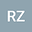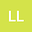loading page

On the Wolff-type Integral System with Negative Exponents
••Ling Li
Nanjing Normal University
Author Profile## Abstract

In this paper, we are concerned with the positive continuous entire solutions of the Wolff-type integral system \begin{equation*} \left\{ \begin{array}{ll} &u(x) =C_{1}(x)W_{\beta,\gamma} (v^{-q})(x), \\[3mm] &v(x) =C_{2}(x)W_{\beta,\gamma} (u^{-p})(x), \end{array} \right. \end{equation*} where $n\geq1$, $\min\{p,q\}>0$, $\gamma>1$, $\beta>0$ and $\beta\gamma\neq n$. In addition, $C_{i}(x) \ (i=1,2)$ are some double bounded functions. If $\beta\gamma\in (0,n)$, the Serrin-type condition is critical for existence of the positive solutions for some double bounded functions $C_{i}(x)$ $(i=1,2)$. Such an integral equation system is related to the study of the $\gamma$-Laplace system and $k$-Hessian system with negative exponents. Estimated by the integral of the Wolff type potential, we obtain the asymptotic rates and the integrability of positive solutions, and studied whether the radial solutions exist.

#### Peer review status:UNDER REVIEW

28 Jan 2021Submitted to Mathematical Methods in the Applied Sciences
28 Jan 2021Assigned to Editor
28 Jan 2021Submission Checks Completed
04 Feb 2021Reviewer(s) Assigned